Equilibria of Other Reaction Classes

# 92 Lewis Acids and Bases

### Learning Objectives

By the end of this section, you will be able to:

• Explain the Lewis model of acid-base chemistry
• Write equations for the formation of adducts and complex ions
• Perform equilibrium calculations involving formation constants

In 1923, G. N. Lewis proposed a generalized definition of acid-base behavior in which acids and bases are identified by their ability to accept or to donate a pair of electrons and form a coordinate covalent bond.

A coordinate covalent bond (or dative bond) occurs when one of the atoms in the bond provides both bonding electrons. For example, a coordinate covalent bond occurs when a water molecule combines with a hydrogen ion to form a hydronium ion. A coordinate covalent bond also results when an ammonia molecule combines with a hydrogen ion to form an ammonium ion. Both of these equations are shown here.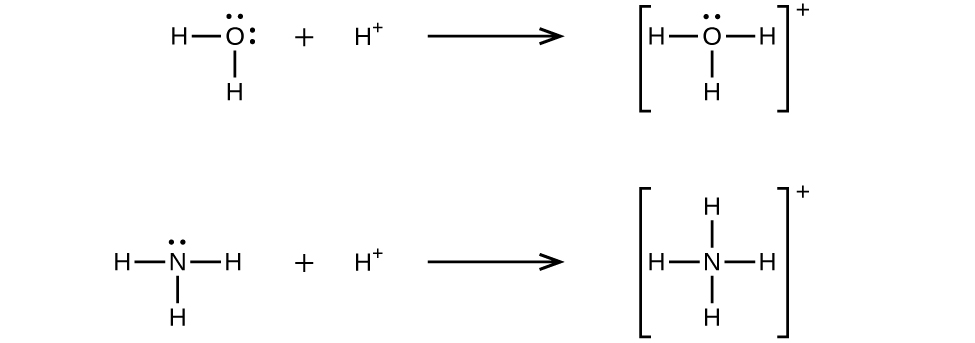Reactions involving the formation of coordinate covalent bonds are classified as Lewis acid-base chemistry. The species donating the electron pair that compose the bond is a Lewis base, the species accepting the electron pair is a Lewis acid, and the product of the reaction is a Lewis acid-base adduct. As the two examples above illustrate, Brønsted-Lowry acid-base reactions represent a subcategory of Lewis acid reactions, specifically, those in which the acid species is H+. A few examples involving other Lewis acids and bases are described below.

The boron atom in boron trifluoride, BF3, has only six electrons in its valence shell. Being short of the preferred octet, BF3 is a very good Lewis acid and reacts with many Lewis bases; a fluoride ion is the Lewis base in this reaction, donating one of its lone pairs: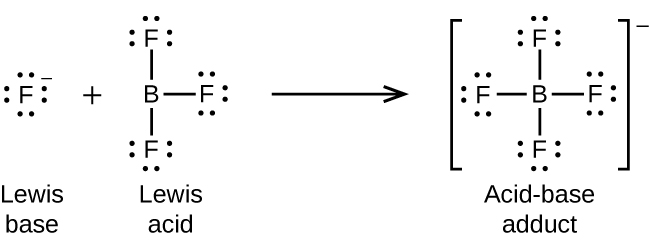In the following reaction, each of two ammonia molecules, Lewis bases, donates a pair of electrons to a silver ion, the Lewis acid: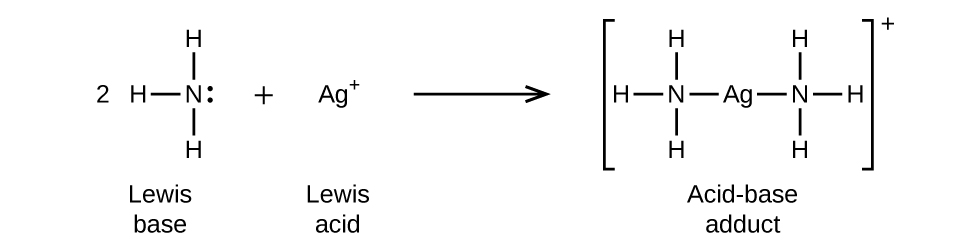Nonmetal oxides act as Lewis acids and react with oxide ions, Lewis bases, to form oxyanions: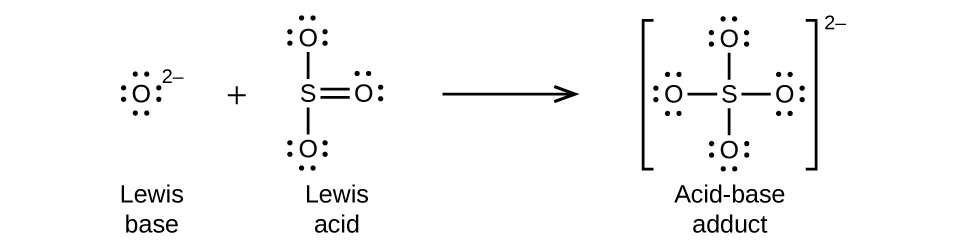Many Lewis acid-base reactions are displacement reactions in which one Lewis base displaces another Lewis base from an acid-base adduct, or in which one Lewis acid displaces another Lewis acid: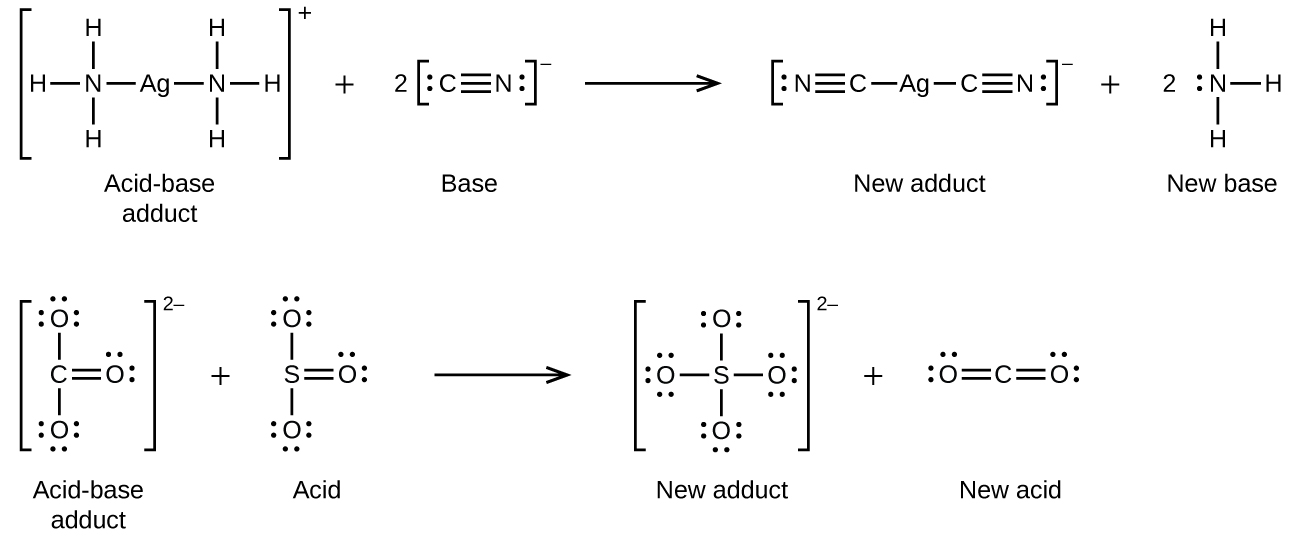Another type of Lewis acid-base chemistry involves the formation of a complex ion (or a coordination complex) comprising a central atom, typically a transition metal cation, surrounded by ions or molecules called ligands. These ligands can be neutral molecules like H2O or NH3, or ions such as CN or OH. Often, the ligands act as Lewis bases, donating a pair of electrons to the central atom. These types of Lewis acid-base reactions are examples of a broad subdiscipline called coordination chemistry—the topic of another chapter in this text.

The equilibrium constant for the reaction of a metal ion with one or more ligands to form a coordination complex is called a formation constant (Kf) (sometimes called a stability constant). For example, the complex ion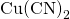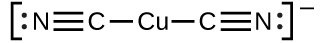is produced by the reactionThe formation constant for this reaction is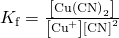Alternatively, the reverse reaction (decomposition of the complex ion) can be considered, in which case the equilibrium constant is a dissociation constant (Kd). Per the relation between equilibrium constants for reciprocal reactions described, the dissociation constant is the mathematical inverse of the formation constant, Kd = Kf–1. A tabulation of formation constants is provided in Appendix K.

As an example of dissolution by complex ion formation, let us consider what happens when we add aqueous ammonia to a mixture of silver chloride and water. Silver chloride dissolves slightly in water, giving a small concentration of Ag+ ([Ag+] = 1.310–5M):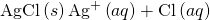However, if NH3 is present in the water, the complex ion,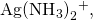can form according to the equation:

Dissociation of a Complex Ion Calculate the concentration of the silver ion in a solution that initially is 0.10 M with respect to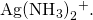Solution Applying the standard ICE approach to this reaction yields the following: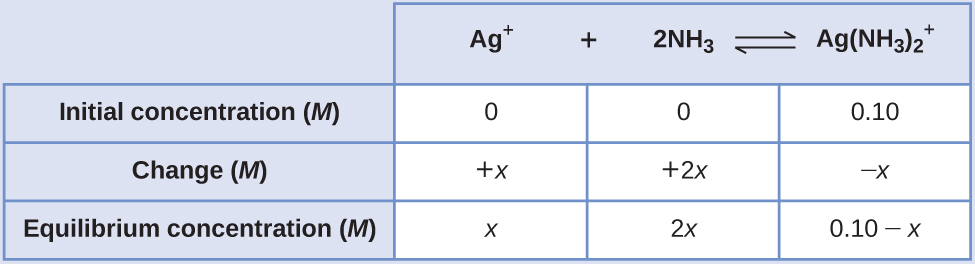Substituting these equilibrium concentration terms into the Kf expression gives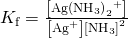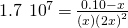The very large equilibrium constant means the amount of the complex ion that will dissociate, x, will be very small. Assuming x << 0.1 permits simplifying the above equation: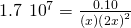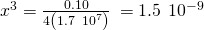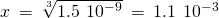Because only 1.1% of the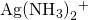dissociates into Ag+ and NH3, the assumption that x is small is justified.

Using this value of x and the relations in the above ICE table allows calculation of all species’ equilibrium concentrations: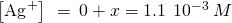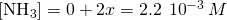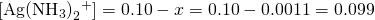The concentration of free silver ion in the solution is 0.0011 M.

Check Your Learning Calculate the silver ion concentration, [Ag+], of a solution prepared by dissolving 1.00 g of AgNO3 and 10.0 g of KCN in sufficient water to make 1.00 L of solution. (Hint: Because Kf is very large, assume the reaction goes to completion then calculate the [Ag+] produced by dissociation of the complex.)

Answer:

2.510–22M

### Key Concepts and Summary

A Lewis acid is a species that can accept an electron pair, whereas a Lewis base has an electron pair available for donation to a Lewis acid. Complex ions are examples of Lewis acid-base adducts and comprise central metal atoms or ions acting as Lewis acids bonded to molecules or ions called ligands that act as Lewis bases. The equilibrium constant for the reaction between a metal ion and ligands produces a complex ion called a formation constant; for the reverse reaction, it is called a dissociation constant.

### Chemistry End of Chapter Exercises

Even though Ca(OH)2 is an inexpensive base, its limited solubility restricts its use. What is the pH of a saturated solution of Ca(OH)2?

Under what circumstances, if any, does a sample of solid AgCl completely dissolve in pure water?

when the amount of solid is so small that a saturated solution is not produced

Explain why the addition of NH3 or HNO3 to a saturated solution of Ag2CO3 in contact with solid Ag2CO3 increases the solubility of the solid.

Calculate the cadmium ion concentration, [Cd2+], in a solution prepared by mixing 0.100 L of 0.0100 M Cd(NO3)2 with 1.150 L of 0.100 NH3(aq).

810–5M

Explain why addition of NH3 or HNO3 to a saturated solution of Cu(OH)2 in contact with solid Cu(OH)2 increases the solubility of the solid.

Sometimes equilibria for complex ions are described in terms of dissociation constants, Kd. For the complex ion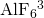the dissociation reaction is: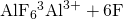and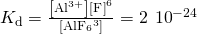Calculate the value of the formation constant, Kf, for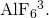51023

Using the value of the formation constant for the complex ion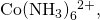calculate the dissociation constant.

Using the dissociation constant, Kd = 7.810–18, calculate the equilibrium concentrations of Cd2+ and CN in a 0.250-M solution of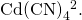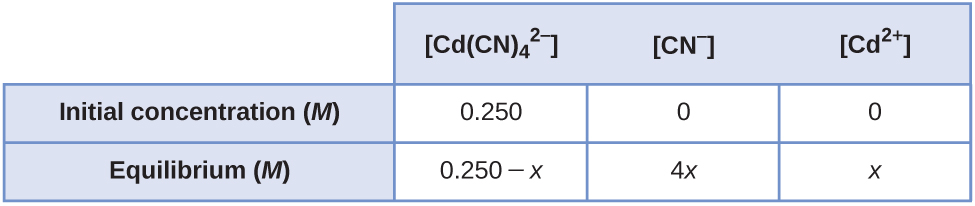[Cd2+] = 9.510–5M; [CN] = 3.810–4M

Using the dissociation constant, Kd = 3.410–15, calculate the equilibrium concentrations of Zn2+ and OH in a 0.0465-M solution of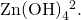Using the dissociation constant, Kd = 2.210–34, calculate the equilibrium concentrations of Co3+ and NH3 in a 0.500-M solution of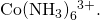[Co3+] = 3.010–6M; [NH3] = 1.810–5M

Using the dissociation constant, Kd = 110–44, calculate the equilibrium concentrations of Fe3+ and CN in a 0.333 M solution of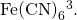Calculate the mass of potassium cyanide ion that must be added to 100 mL of solution to dissolve 2.010–2 mol of silver cyanide, AgCN.

1.3 g

Calculate the minimum concentration of ammonia needed in 1.0 L of solution to dissolve 3.010–3 mol of silver bromide.

A roll of 35-mm black and white photographic film contains about 0.27 g of unexposed AgBr before developing. What mass of Na2S2O3·5H2O (sodium thiosulfate pentahydrate or hypo) in 1.0 L of developer is required to dissolve the AgBr as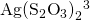(Kf = 4.71013)?

0.79 g

We have seen an introductory definition of an acid: An acid is a compound that reacts with water and increases the amount of hydronium ion present. In the chapter on acids and bases, we saw two more definitions of acids: a compound that donates a proton (a hydrogen ion, H+) to another compound is called a Brønsted-Lowry acid, and a Lewis acid is any species that can accept a pair of electrons. Explain why the introductory definition is a macroscopic definition, while the Brønsted-Lowry definition and the Lewis definition are microscopic definitions.

Write the Lewis structures of the reactants and product of each of the following equations, and identify the Lewis acid and the Lewis base in each:

(a)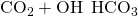(b)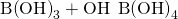(c)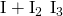(d)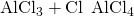(use Al-Cl single bonds)

(e)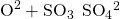(a)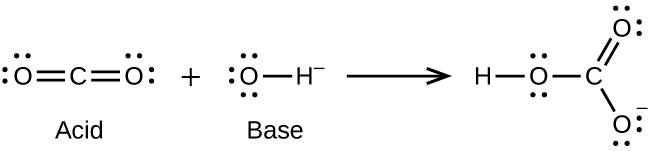(b)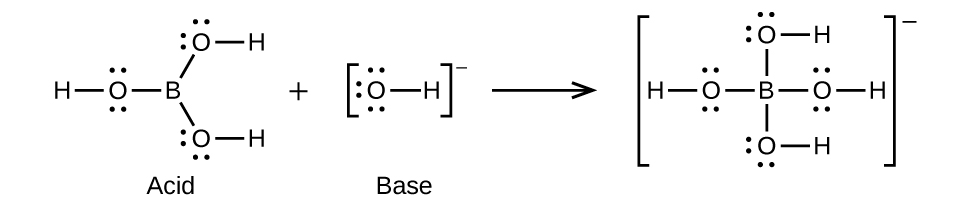(c)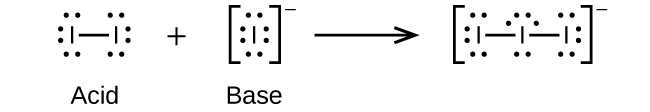(d)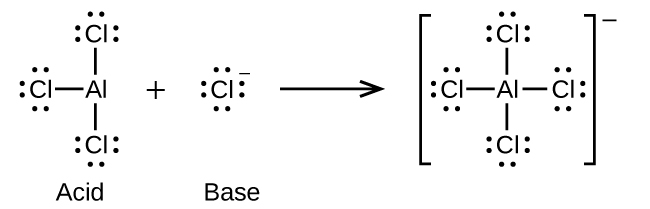(e)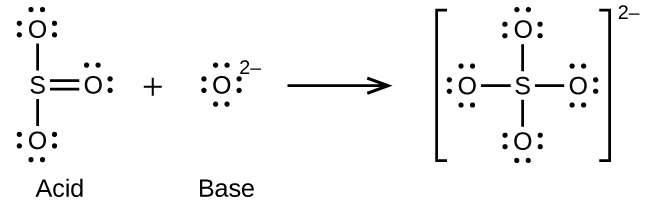Write the Lewis structures of the reactants and product of each of the following equations, and identify the Lewis acid and the Lewis base in each:

(a)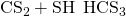(b)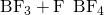(c)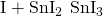(d)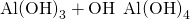(e)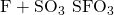Using Lewis structures, write balanced equations for the following reactions:

(a)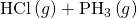(b)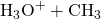(c)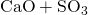(d)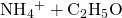(a)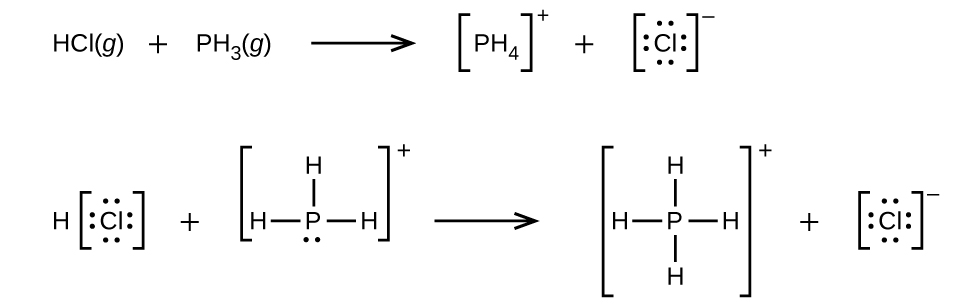(b)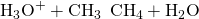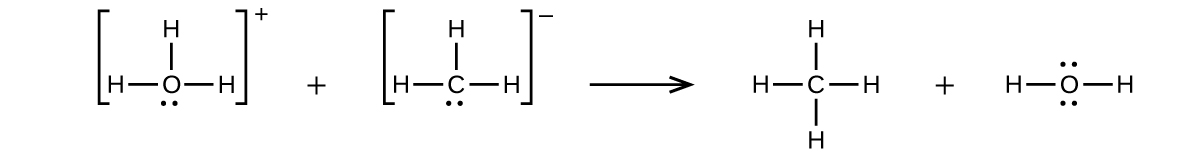(c)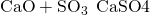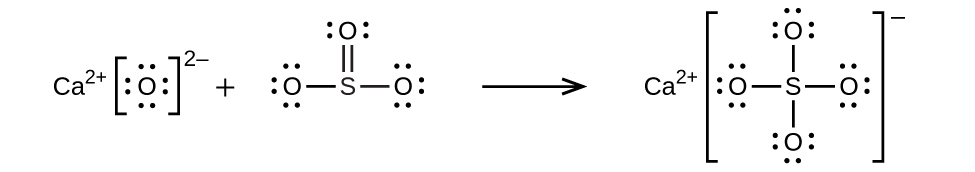(d)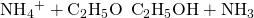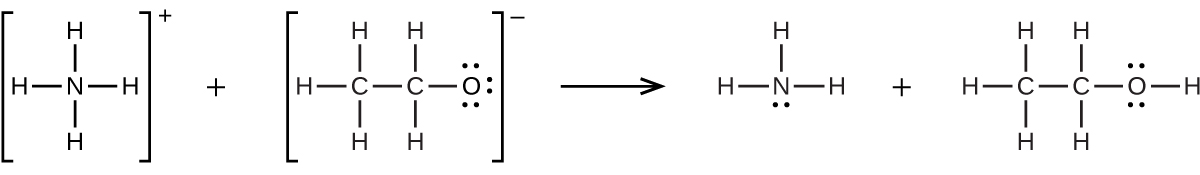Calculate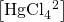in a solution prepared by adding 0.0200 mol of NaCl to 0.250 L of a 0.100-M HgCl2 solution.

In a titration of cyanide ion, 28.72 mL of 0.0100 M AgNO3 is added before precipitation begins. [The reaction of Ag+ with CN goes to completion, producing the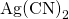complex.] Precipitation of solid AgCN takes place when excess Ag+ is added to the solution, above the amount needed to complete the formation of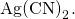How many grams of NaCN were in the original sample?

0.0281 g

What are the concentrations of Ag+, CN, andin a saturated solution of AgCN?

In dilute aqueous solution HF acts as a weak acid. However, pure liquid HF (boiling point = 19.5 °C) is a strong acid. In liquid HF, HNO3 acts like a base and accepts protons. The acidity of liquid HF can be increased by adding one of several inorganic fluorides that are Lewis acids and accept F ion (for example, BF3 or SbF5). Write balanced chemical equations for the reaction of pure HNO3 with pure HF and of pure HF with BF3.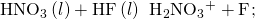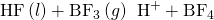The simplest amino acid is glycine, H2NCH2CO2H. The common feature of amino acids is that they contain the functional groups: an amine group, –NH2, and a carboxylic acid group, –CO2H. An amino acid can function as either an acid or a base. For glycine, the acid strength of the carboxyl group is about the same as that of acetic acid, CH3CO2H, and the base strength of the amino group is slightly greater than that of ammonia, NH3.

(a) Write the Lewis structures of the ions that form when glycine is dissolved in 1 M HCl and in 1 M KOH.

(b) Write the Lewis structure of glycine when this amino acid is dissolved in water. (Hint: Consider the relative base strengths of the –NH2 and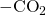groups.)

Boric acid, H3BO3, is not a Brønsted-Lowry acid but a Lewis acid.

(a) Write an equation for its reaction with water.

(b) Predict the shape of the anion thus formed.

(c) What is the hybridization on the boron consistent with the shape you have predicted?

(a)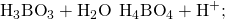(b) The electronic and molecular shapes are the same—both tetrahedral. (c) The tetrahedral structure is consistent with sp3 hybridization.

### Glossary

complex ion
ion consisting of a central atom surrounding molecules or ions called ligands via coordinate covalent bonds
coordinate covalent bond
(also, dative bond) covalent bond in which both electrons originated from the same atom
dissociation constant
(Kd) equilibrium constant for the decomposition of a complex ion into its components
formation constant
(Kf) (also, stability constant) equilibrium constant for the formation of a complex ion from its components
Lewis acid
any species that can accept a pair of electrons and form a coordinate covalent bond
Lewis acid-base adduct
compound or ion that contains a coordinate covalent bond between a Lewis acid and a Lewis base
Lewis acid-base chemistry
reactions involving the formation of coordinate covalent bonds
Lewis base
any species that can donate a pair of electrons and form a coordinate covalent bond
ligand
molecule or ion acting as a Lewis base in complex ion formation; bonds to the central atom of the complex

## LicenseChemistry 2e by OSCRiceUniversity is licensed under a Creative Commons Attribution 4.0 International License, except where otherwise noted.